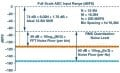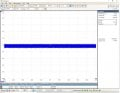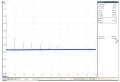# Noise floor measurement with Pico 4262

#### Integrole

Joined Apr 15, 2021
1
Hi all,

I would like to measure the noise floor of several amplifiers in the 10Hz-10 MHz band.
I would like to use a pico 4262 ( Dual channel oscilloscope / spectrum analyzer, 16-bit resolution, Low distortion (96 dB SFDR), Low noise (8.5 µV RMS), 5 MHz bandwidth)In FFT mode, the noise displayed by the pico scope is the orange curve (that is the FFT Noise Floor).

* To get the RMS noise level ( in uV), I add 10log(M/2) (Process gain, more info https://www.picotech.com/support/viewtopic.php?t=39442&start=15 ). M is the number of FFT Bin points. To get Vrms value, I use the calculator http://www.sengpielaudio.com/calculator-db-volt.htm

* To get the noise floor ( blue curve) ( per Hz), I subtract 10log(fs/2)- 10log(M/2) = 10log(fs/M).

I measure the noise floor of a certain amplifier in two ways ( time mode and fft mode):
Gain=1
BW = 5 MHz
Sample rate ( Fs) : 10 MHz
Range = 10 mV
M = 1048576 bin points
Couplage : AC

My goal is to obtain the RTI Voltage Noise spectral density ( in nV/sqrt(Hz) )

Time mode :The peak to peak voltage is about Vpp = 1.1 mV (measured by hand, I don't trust the values displayed).
So Vrms noise = Vpp/6. https://www.allaboutcircuits.com/te...istribution-noise-rms-peak-to-peak-value-PSD/
So we have Vrms = 180 uV. We want to get the RTI Voltage Noise spectral density , so we divide by the square root of the band:

VRTI = Vrms / sqrt( (pi/2)*5MHz) = 6.4E-8 = 64 nV/sqrt(Hz).

FFT mode ( with M = 1048576 points bin)

I use Average mode ( Blackman windows) :We measure -136 dBV approximately ( orange curve of the first graph).

* To get the Noise Floor ( blue curve of the first graph)., I subtract by 10log(Fs/M) = 10log(10E6/1048576) = 10 Hz so 10 dB.
The result is -146dBV and therefore about 45nV/sqrt(Hz) with the calculator.

Another way to do it:
* To get the RMS value, we add the process gain ( 10log(1048576/2) = 57 dB.
The result is -79 dBV RMS and therefore 112 uV RMS with the calculator.
We want to get the RTI Voltage, so we divide by the square root of the band:
VRTI = Vrms / sqrt( (pi/2)*5MHz) = 112E-6 / sqrt( (3.14/2)*5MHz) = 40 nV/sqrt(Hz)

This is what I get, does the measurement method seem correct to you?
Any commentary ? Indeed, noise measurement is a rather complex measurement, and it is easy to measure anything!

Thank you very much.

#### Deleted member 115935

Joined Dec 31, 1969
0
pico have an active forum and tech support site,
why not ask them how they test / define the numbers ?